Select Page

# 12 Science Maths CBSE Continuity and Differentiability Solutions for MCQ in English

12 Science Maths CBSE Continuity and Differentiability Solutions for MCQ in English to enable students to get Solutions in a narrative video format for the specific question.

Expert Teacher provides 12 Science Maths CBSE Continuity and Differentiability Solutions for MCQ through Video Solutions in English language. This video solution will be useful for students to understand how to write an answer in exam in order to score more marks. This teacher uses a narrative style for a question from Continuity and Differentiability not only to explain the proper method of answering question, but deriving right answer too.

Please find the question below and view the Solution in a narrative video format.

Question:

Solution Video in English:

You can select video Solutions from other languages also. Please check Solutions in ( Hindi )

## Similar Questions from CBSE, 12th Science, Maths, Continuity and Differentiability

Question 1 :  Find the second order derivative of the function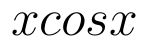. (View Answer Video)

Question 2 : Differentiate the function w.r.t.x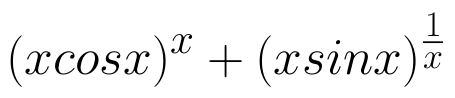. (View Answer Video)

Question 3 : If x and y are connected parametrically by the equation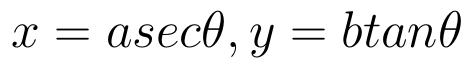, without eliminating the parameter, find. (View Answer Video)

Question 4 :  Find the second order derivative of the function. (View Answer Video)

Question 5 : Differentiate w.r.t.x the function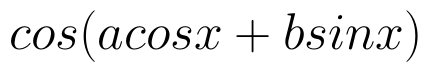, for some constant a and b. (View Answer Video)

### Relations and Functions

Question 1 :  If f is the greatest integer function and g is the modulus function . Write the value of g o f(-1/3) – f o g ( -1/3 ) . (View Answer Video)

Question 2 : Letbe defined as f(x) = 3x. Choose the correct answer. (View Answer Video)

Question 4 :  Let R be the relation on the set {1, 2, 3, 4} given by R = {(1, 2), (2, 2), (1, 1), (4, 4), (1, 3), (3,3), (3,2)}. then R is, (View Answer Video)

Question 5 : Let * be the binary operation on N given by a * b = LCM of a and b. Find the identity of * in N. (View Answer Video)

### Application of Derivatives

Question 1 : The total revenue (in Rs) received from the sale of 'x' units of a product is given by :
R(x) =3x2+36x+5.
FInd the marginal revenue when x=5, where by marginal revenue we mean the rate of change of total revenue with respect to the number of items sold at an instant. (View Answer Video)

Question 2 : The line y=mx+1 is a tangent to the curve if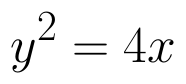the value of m is ________. (View Answer Video)

Question 3 : The length x of a rectangle is decreasing at the rate of 5cm/minute. and width y is increasing at the rate of 4cm/minute. When x=8cm and y=6 cm, find the rate of changes of:

Question 4 : The maximum value of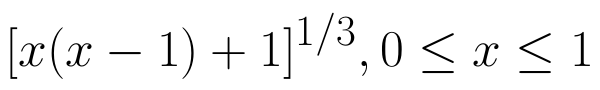is (View Answer Video)

Question 5 : The maximum value of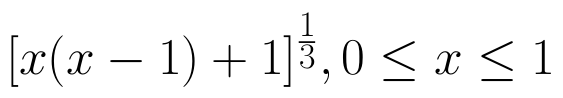is, (View Answer Video)

### Probability

Question 1 :  A coin is tossed three times. Find P(F/E), where E : at most two tails and F : atleast one tail.  (View Answer Video)

Question 2 : A couple has 2 children. Find the probability that both are boys, if it is known that one of them is a boy.  (View Answer Video)

Question 3 : If E and F be two evets such that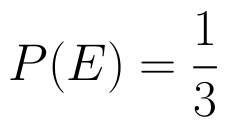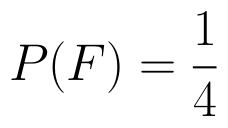, find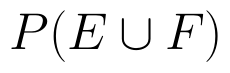if E and F are independent events. (View Answer Video)

Question 4 :  A and B throw a pair of dice alternatively, till one of them gets a total of 10 and wins the game. Find their respective probabilities of winning, if A starts first.   (View Answer Video)

Question 5 : If P(A)=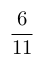, P(B)=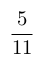and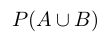=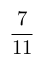, find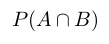. (View Answer Video)